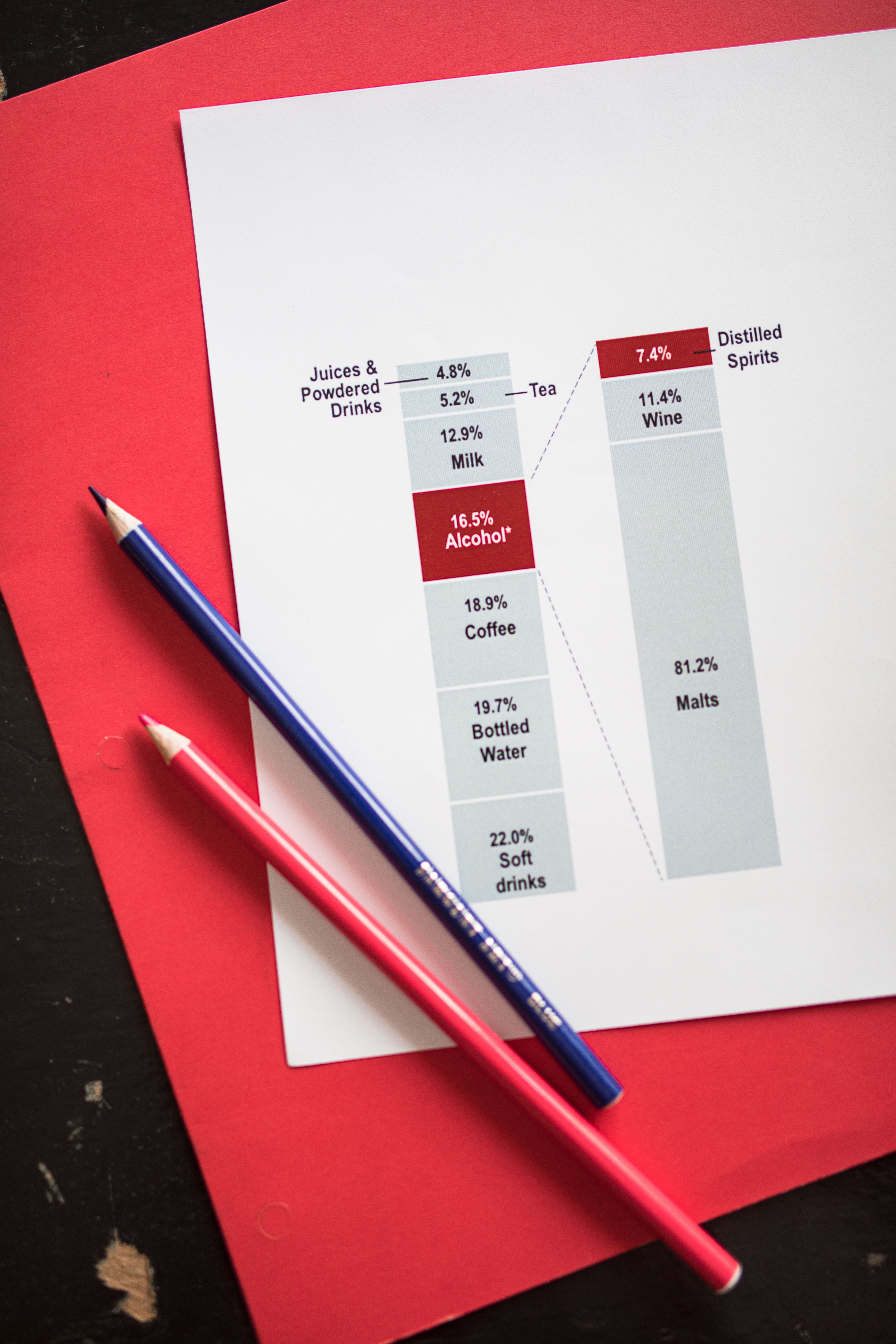# What do you learn in elementary statistics?

What Do You Learn in Elementary Statistics?

# What Do You Learn in Elementary Statistics?Statistics is a branch of mathematics which deals with collecting, analyzing, and interpreting data. It is present in our daily lives, from marking attendance to calculating household expenses to predicting the weather. Statistics is crucial in our society and economy as it allows us to make informed decisions by understanding the data presented to us. In this article, we will discuss what you learn in elementary statistics.

## FAQs

### What are the topics included in elementary statistics?

Elementary statistics is a branch of statistics that covers the basics of statistical analysis. Topics covered in this course include:

• Descriptive statistics
• Probability
• Distributions
• Hypothesis testing
• Inferences
• Correlation
• Regression### What is descriptive statistics?

Descriptive statistics are used to describe or summarize a set of data. This branch of statistics can be used to calculate measures of central tendency, such as mean, mode, and median. It can also calculate measures of variability, such as range, variance, and standard deviation. Descriptive statistics can help us gain insight into the data and understand its characteristics, such as distribution and outliers.

### What is probability in statistics?

Probability is a branch of mathematics that deals with the likelihood of an event occurring. In statistics, probability is used to predict the likelihood of an outcome based on the available data. Probability helps statisticians make better decisions by understanding the chances of certain events happening. For example, in a medical study, data analysts can predict the probability of a certain disease occurring in certain populations based on the prevalence of risk factors.### What are distributions in statistics?

Distributions are used to represent the frequency of data values in a dataset. A distribution gives an idea of how common or uncommon different values are in the dataset. One of the most common distributions is the normal distribution which has a bell-shaped curve. The normal distribution is used in many statistical analyses because it is very common in nature, such as height, weight, and test scores.

### What is hypothesis testing in statistics?

Hypothesis testing is a statistical process used to determine whether a hypothesis is true or false based on a sample of data. This process is used in research studies to compare two or more groups and determine if there is a statistically significant difference between them. Statisticians formulate a hypothesis and test it against sample data to determine if it is accurate or not. If the hypothesis is accepted, it means that the data collected supports the hypothesis, and if it is rejected, then it means that there is insufficient data to support the hypothesis.### What is correlation in statistics?

Correlation is used to measure the strength and direction of a relationship between two variables. A correlation coefficient is used to measure the level of correlation, where a value of 0 means no correlation, and a value of 1 means a perfect positive correlation. A value of -1 means a perfect negative correlation. Understanding the correlation between variables helps researchers and analysts to make informed decisions.

### What is regression in statistics?

Regression is used to model the relationship between a dependent variable and one or more independent variables. The regression analysis helps to quantify the relationship between the variables and make predictions. There are several types of regression, such as linear regression, which is used to model a linear relationship between the dependent and independent variables.